CLAT  >  Introduction to Heat Transfer

# Introduction to Heat Transfer Notes | Study Current Affairs & General Knowledge - CLAT

## Document Description: Introduction to Heat Transfer for CLAT 2022 is part of Introduction to Heat Transfer for Current Affairs & General Knowledge preparation. The notes and questions for Introduction to Heat Transfer have been prepared according to the CLAT exam syllabus. Information about Introduction to Heat Transfer covers topics like and Introduction to Heat Transfer Example, for CLAT 2022 Exam. Find important definitions, questions, notes, meanings, examples, exercises and tests below for Introduction to Heat Transfer.

Introduction of Introduction to Heat Transfer in English is available as part of our Current Affairs & General Knowledge for CLAT & Introduction to Heat Transfer in Hindi for Current Affairs & General Knowledge course. Download more important topics related with Introduction to Heat Transfer, notes, lectures and mock test series for CLAT Exam by signing up for free. CLAT: Introduction to Heat Transfer Notes | Study Current Affairs & General Knowledge - CLAT
 1 Crore+ students have signed up on EduRev. Have you?

What is Heat?

Heat is Energy in Transit. Recall the First law from Thermodynamics.

ΔU = Q - W

What did we mean by all the terms?

• What is ΔU ?
• What is Q ?
• What is W ?

What is Heat Transfer?

Heat transfer is the transfer of Heat effected by a temperature difference. However, in contrast to what we may have done in Thermodynamics, we are concerned with the rate of heat transfer. For that, we need a different energy balance, one that accounts for the rate of transfer and production, a general energy balance.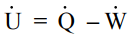Examples of Heat Transfer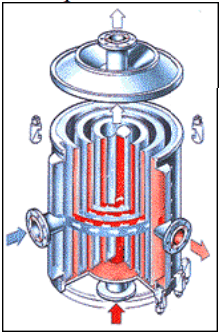• Pasteurizing milk
• Energy loss from a house by conduction through a window
• Melting of a polymer
• Cooling an automobile engine
• Cooling or heating a stream in a chemical process
• Condensing vapor leaving a distillation tower
• Solar heating of the earth
• Radiational cooling of a pond
• Air-conditioning
• Temperature control of the human body
• Ablational cooling of a space shuttle on reentry

Modes of Heat Transfer

• Conduction
• Convection

Conduction is the thermal equivalent of diffusion. In gases, energy is exchanged between molecules in collisions. You will recall from Thermodynamics that a measure of the molecular motion and the energy exchange is the temperature and that conduction is the transfer of energy from the more energetic molecules to the less energetic. In the presence of a temperature gradient, energy is then transferred from the high temperature ( more energetic) to the lower temperature(less energetic). For liquids and solids, the example is not as clear. Conduction in liquids the collisions are more frequent and not as energetic and in solids transfer is by vibrations, for example, in a crystal lattice.

The constitutive equation describing the phenomenon is Fourier’s law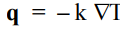What we mean by the notation is the following:

q = q1e1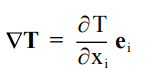Conduction in one-dimension

To give you some notion of what I mean, examine a simple onedimensional conduction problem.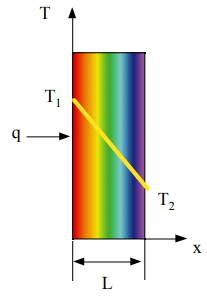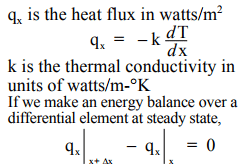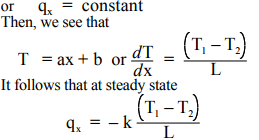Convection

Convection in heat transfer is a process which involves the diffusion of heat and the advection of energy by flow.

The process can be described by Newton’s Law of Cooling

q . n = – h (T – Tb )where h is the heat transfer coefficient as watts/m2 -°K

Typical Values of Heat Transfer Coefficients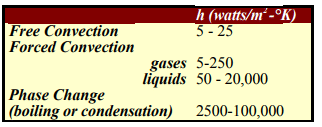What is the relation between h expressed in English units and SI units ?

In SI units , h = 10 watts/m2 -°K

In English units, h = 1.7612 BTU/hr-ft2 -°F

Quick estimates for conversion changes, divide watts/m2 -°K by 6 to get BTU/hr-ft2 -°F

Thermal radiation is energy emitted by matter that is at a finite temperature. Emission occurs not only from solids, but from gases and liquids. The energy is carried by electromagnetic waves, originating at the expense of the internal energy of the matter. Conduction and convection depend on the presence of an intermediary. Radiation does not !

The normal component of heat flux emitted by a surface is given by the Stefan-Boltzmann’s Law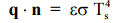where ε is the emissivity σ is the Stefan-Boltzman constant The emissivity is the ratio of the energy emitted by the real surface compared to that emitted by an ideal surface (a black body).

It is dimensionless. σ = 5.67 x 10-8 watts/m2 -K4 and T is in degrees Kelvin. The net rate of energy transfer between two surfaces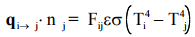The view factor, Fij , depends on the distance R between and the orientation of the two surfaces. We will discuss Radiation in the last several weeks of the course.

Problem Solving

Your textbook outlines a "stock" procedure for addressing, formulating and solving problems in Heat Transfer. In my view, it is a sound technique and one that I would ask that you follow. It involves several steps, each with a prescribed formula. What folloiws is the outline.

Problem Statement The statement of the problem and the results required

Solution

1. Known

Read the problem carefully, then state briefly and concisely what is know about the problem. This is not a simple restaement of the problem.

2. Find

Briefly and concisely state the results that are required.

3. Schematic

Draw a picture (schematic) of the system. Identify and label the heat transfer processes and the relevant boundaries of the system (control surfaces)

4. Assumptions

List all the relevant assumptions you will use in formulating the problem.

5. Properties

Compile the property valued for all the calculations to follow and identify the sources of the data.

6. Analysis

Apply the appropriate conservation laws and appropriate constitutive equations ( rate laws). Develop the analysis as completely as you can without substituting numerical values. If it is feasible, make the equations dimensionless. Finally complete the calculation with numerical values.

Discuss the results. This should include the key conclusions. Were the initial assumptions valid ? What effect would there be if they were not? How sensitive is your solution to the parameters?

Measurement of Thermal Conductivity

A Design Problem

Statement of the Problem

Measure the conductivity of the metal in a metal rod connected to a constant temperature sink (an ice bath) Water flows through a well-mixed reservoir. In enters at Th1 and leaves at the same temperature as it is in the water reservoir, Th2. The rod, the two reservoirs are insulated from the surroundings. Derive a relationship for the thermal conductivity and recommend a flow rate for the water.

Solution

1. Known

The heat lost by the fluid in the lower reservoir is transferred to the ice batch through the rod. The temperature is known for the ce bath. The water flow is measured and known, and we measure the water temperatures in and out of the reservoir.

2. Find Derive a relationship for the thermal conductivity and recommend a flow rate for the water.

3. Schematic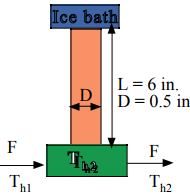4. Assumptions

There are a number of assumptions in this energy balance. What are they?

5. Properties

Some are given in the schematic, but what should we list ?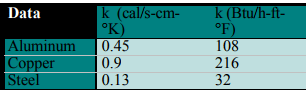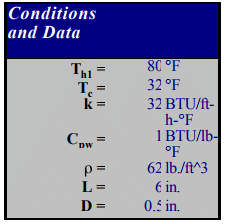6. Analysis

Energy balance on the rod Heat exchanged from the water = heat transferred across the rod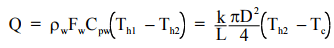We did not state the constitutive relations . What are they? There are a number of assumptions in this energy balance. What are they?

We can solve for the thermal conductivity , k.. The result gives us an equation by which we might better design the experiment.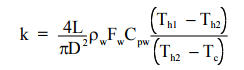The dimensions, L and D are fixed as are all the variables save Th1 and Fw . What are the best choices for these variables?

Dimensionless form for the solution.

We can group parameters as θ, so that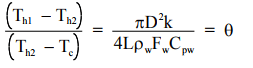and the temperature rise can be expressed simply as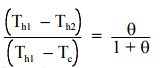If we calculate the temperature rise as a function of flow rate we can obtain the following table.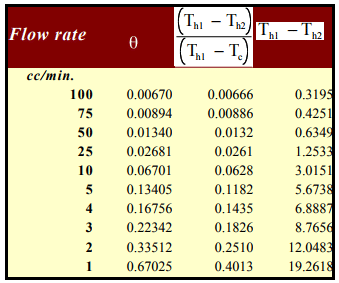In an experiment we can measure temperature no more precisely that 0.1 °F so that the highest flow rate would be 5 ml/min.

Some experimental questions

• How precise can you control and measure flow rates?
• How good is the well-stirred assumption?
• How precise are the temperature measurements?
• Given the precision of measurement, what is the precision of the estimation of the thermal conductivity?
The document Introduction to Heat Transfer Notes | Study Current Affairs & General Knowledge - CLAT is a part of the CLAT Course Current Affairs & General Knowledge.
All you need of CLAT at this link: CLAT

## Current Affairs & General Knowledge

128 videos|580 docs|35 tests
 Use Code STAYHOME200 and get INR 200 additional OFF

## Current Affairs & General Knowledge

128 videos|580 docs|35 tests

Track your progress, build streaks, highlight & save important lessons and more!

,

,

,

,

,

,

,

,

,

,

,

,

,

,

,

,

,

,

,

,

,

;